# 🐢 B4XTurtle - Examples for teachers and parents

Status
Not open for further replies.

#### Erel

Staff member
The purpose of this thread is to build a collection of examples that will help you, the teacher or parent, learn how to use and teach with B4XTurtle library.

If you have any question, feedback or request then please post it here: https://www.b4x.com/android/forum/threads/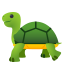---b4xturtle-examples-for-teachers-discuss-here.115896/

The attached projects include a suggested template. It is clean and simple and looks like this: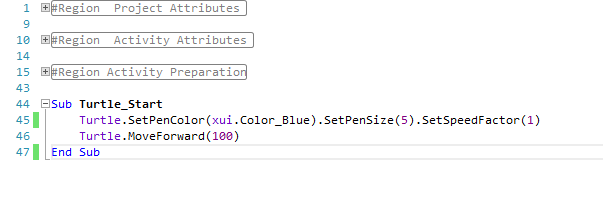Some of the examples are based on: https://personal.utdallas.edu/~veerasam/logo/

The examples are not listed in any special order.
Feel free to use this examples in any way you like.

B4XTurtle is an internal library in B4J v8.3+.

#### Attachments

• 9.3 KB Views: 162
• 110.3 KB Views: 72
Last edited:
••jose luis gudino, JukkaHo, maXim and 5 others

#### Erel

Staff member
Drawing a square: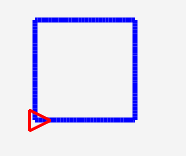Implementation #1:

B4X:
``````Sub Turtle_Start
Turtle.SetPenColor(xui.Color_Blue).SetPenSize(5).SetSpeedFactor(1)
Turtle.MoveForward(100).TurnLeft(90)
Turtle.MoveForward(100).TurnLeft(90)
Turtle.MoveForward(100).TurnLeft(90)
Turtle.MoveForward(100).TurnLeft(90)
End Sub``````
Implementation #2:
With a For loop
B4X:
``````Sub Turtle_Start
Turtle.SetPenColor(xui.Color_Blue).SetPenSize(5).SetSpeedFactor(1)
For i = 1 To 4
Turtle.MoveForward(100).TurnLeft(90)
Next
End Sub``````
Implementation #3:
Reusable sub where the edge size is given as a parameter.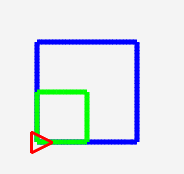B4X:
``````Sub Turtle_Start
Turtle.SetPenColor(xui.Color_Blue).SetPenSize(5).SetSpeedFactor(1)
DrawSquare(100)
'and another smaller green square
Turtle.SetPenColor(xui.Color_Green)
DrawSquare(50)
End Sub

Sub DrawSquare (EdgeSize As Float)
For i = 1 To 4
Turtle.MoveForward(EdgeSize).TurnLeft(90)
Next
End Sub``````

Last edited:

#### Erel

Staff member
Drawing a circle: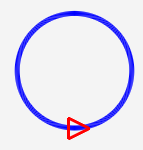Implementation #1:
B4X:
``````Sub Turtle_Start
Turtle.SetPenColor(xui.Color_Blue).SetPenSize(5).SetSpeedFactor(1)
For i = 1 To 360
Turtle.MoveForward(1).TurnLeft(1)
Next
End Sub``````
Implementation #2:
A filled circle: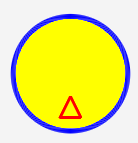B4X:
``````Sub Turtle_Start
Turtle.SetPenColor(xui.Color_Blue).SetPenSize(5).SetSpeedFactor(1)
For i = 1 To 360
Turtle.MoveForward(1).TurnLeft(1)
Next
Turtle.PenUp.TurnLeft(90).MoveForward(20).SetPenColor(xui.Color_Yellow).Fill
End Sub``````
Implementation #3:
A circle with a specific radius:B4X:
``````Sub Turtle_Start
Turtle.SetPenColor(xui.Color_Blue).SetPenSize(5).SetSpeedFactor(1)
Dim radius As Float = 100
Turtle.SetPenColor(0xFFFF00B6) 'right click - color picker
End Sub

Dim DistancePerIteration As Float = 2 * cPI * Radius / 360
For i = 1 To 360
Turtle.MoveForward(DistancePerIteration).TurnLeft(1)
Next
End Sub

Sub DrawSquare (EdgeSize As Float)
For i = 1 To 4
Turtle.MoveForward(EdgeSize).TurnLeft(90)
Next
End Sub``````

#### Erel

Staff member
Drawing a star:Implementation #1:

B4X:
``````Sub Turtle_Start
Turtle.SetPenColor(xui.Color_Blue).SetPenSize(5).SetSpeedFactor(1)
DrawStar (100)
End Sub

Sub DrawStar (EdgeSize As Float)
For i = 1 To 5
Turtle.MoveForward(EdgeSize).TurnRight(144)
Next
End Sub``````
Implementation #2:
With random colors.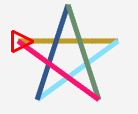B4X:
``````Sub Turtle_Start
Turtle.SetPenColor(xui.Color_Blue).SetPenSize(5).SetSpeedFactor(1)
DrawStar (100)
End Sub

Sub DrawStar (EdgeSize As Float)
For i = 1 To 5
Turtle.SetPenColor(Turtle.RandomColor)
Turtle.MoveForward(EdgeSize).TurnRight(144)
Next
End Sub``````
Implementation #3:
With recursion.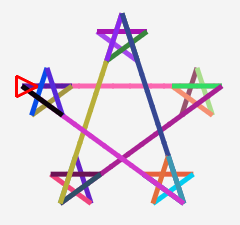B4X:
``````Sub Turtle_Start
Turtle.SetPenColor(xui.Color_Blue).SetPenSize(5).SetSpeedFactor(5)
DrawStar (200)
End Sub

Sub DrawStar (EdgeSize As Float)
If EdgeSize < 50 Then Return
For i = 1 To 5
Turtle.SetPenColor(Turtle.RandomColor)
Turtle.MoveForward(EdgeSize).TurnRight(144)
DrawStar(EdgeSize / 4)
Next
End Sub``````

••maXim, swissmade, Johan Hormaza and 1 other person

#### Erel

Staff member
Drawing random arcs:B4X:
``````Sub Turtle_Start
Turtle.SetPenColor(xui.Color_Blue).SetPenSize(5).SetSpeedFactor(1)
For 1 = 1 To 10
Turtle.SetPenColor(Turtle.RandomColor).SetPenSize(Rnd(2, 10))
Turtle.TurnRight(Rnd(1, 360))
Turtle.Arc(180, Rnd(10, 200))
Next
End Sub``````

#### Erel

Staff member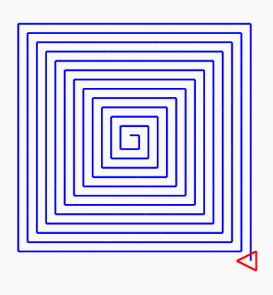B4X:
``````Sub Turtle_Start
Turtle.SetPenColor(xui.Color_Blue).SetPenSize(2).SetSpeedFactor(10)
Dim size As Float = 10
For i = 1 To 50
Turtle.MoveForward(size).TurnRight(90)
size = size + 5
Next
End Sub``````

#### Erel

Staff member
Full circles:B4X:
``````Sub Turtle_Start
Turtle.SetPenColor(xui.Color_Blue).SetPenSize(2).SetSpeedFactor(10)
Dim size As Float = 150
For i = 1 To 8
Turtle.SetPenColor(Turtle.RandomColor).Arc(360, size).Fill
size = size - 20
Next
End Sub``````
Note that the Fill command is a bit slow on mobile devices and the result is not perfect because of antialiasing effects.

Last edited:

#### Erel

Staff member
Growing triangles: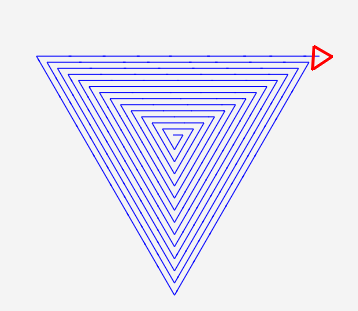B4X:
``````Sub Turtle_Start
Turtle.SetPenColor(xui.Color_Blue).SetPenSize(1).SetSpeedFactor(10)
Dim size As Float = 10
For i = 1 To 40
Turtle.MoveForward(size).TurnRight(120)
size = size + 7
Next
End Sub``````

#### Erel

Staff member
Drawing a flower: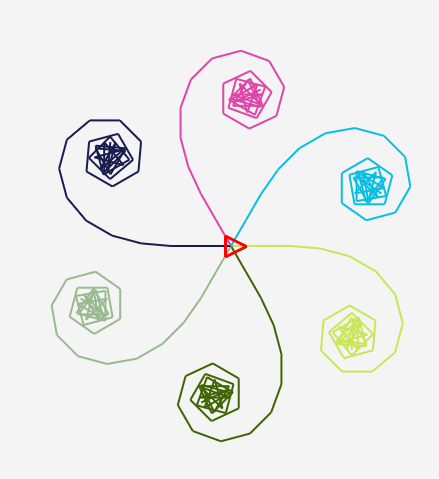B4X:
``````Sub Turtle_Start
Turtle.SetPenColor(xui.Color_Blue).SetPenSize(2).SetSpeedFactor(10)
Dim turn As Float = 0
Do While turn < 360
Turtle.SetPenColor(Turtle.RandomColor)
Dim angle As Float = 0
Do While angle < 180
Turtle.MoveForward(30).TurnRight(angle)
angle = angle + 5
Loop
Turtle.Home
turn = turn + 60
Turtle.TurnRight(turn)
Loop
End Sub``````

#### Erel

Staff member
Squares spiral: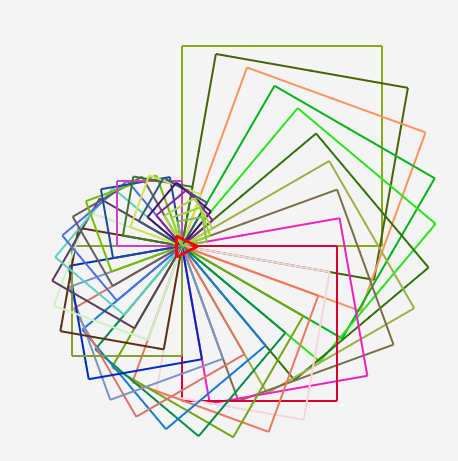B4X:
``````Sub Turtle_Start
Turtle.SetPenColor(xui.Color_Blue).SetPenSize(2).SetSpeedFactor(30)
Dim size As Float = 200
For i = 1 To 36
Turtle.SetPenColor(Turtle.RandomColor)
DrawSquare (size)
Turtle.TurnRight(10)
size = size - 5
Next
End Sub

Sub DrawSquare (EdgeSize As Float)
For i = 1 To 4
Turtle.MoveForward(EdgeSize).TurnLeft(90)
Next
End Sub``````

#### Erel

Staff member
Handling the touch event.

Implementation #1: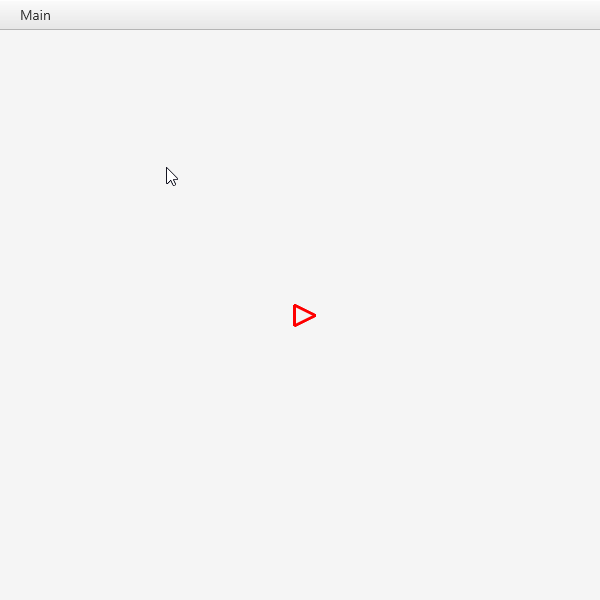B4X:
``````Sub Turtle_Start
Turtle.SetPenColor(xui.Color_Blue).SetPenSize(2).SetSpeedFactor(1)
End Sub

Sub Turtle_Touch (Args As TurtleTouchArgs)
Turtle.MoveTo(Args.X, Args.Y)
End Sub``````
Implementation #2:

This time we call Turtle.Stop in the Touch event. Turtle.Stop is a special command. It clears the actions queue so the turtle immediately stops moving and then with a call to MoveTo starts moving towards the touch point.B4X:
``````Sub Turtle_Start
Turtle.SetPenColor(xui.Color_Blue).SetPenSize(2).SetSpeedFactor(1)
End Sub

Sub Turtle_Touch (Args As TurtleTouchArgs)
Turtle.Stop
Turtle.MoveTo(Args.X, Args.Y)
End Sub``````

••Johan Hormaza and Marc DANIEL

#### Erel

Staff member
Global variables and If condition:

1. Add this line to Process_Globals:
B4X:
``Private PrevX, PrevY As Float``
2.
B4X:
``````Sub Turtle_Touch (Args As TurtleTouchArgs)
Turtle.SetX(Args.X).SetY(Args.Y)
If Args.Down Then
PrevX = Args.X
PrevY = Args.Y
Turtle.MoveForward(2)
Else If Args.Up Then
Turtle.MoveTo(PrevX, PrevY)
End If
End Sub``````
Result: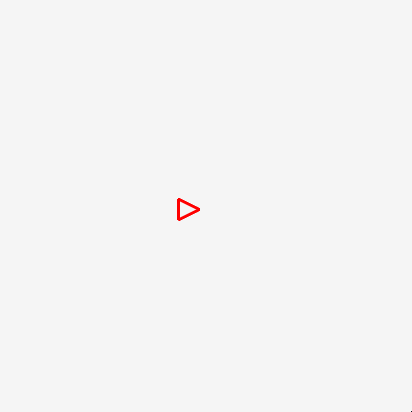#### Erel

Staff member
Tree: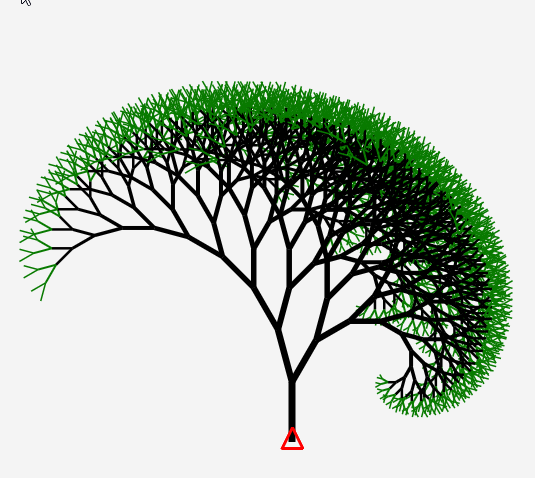B4X:
``````Private Angle1 As Float = 15
Private Angle2 As Float = 30
Private Factor1 As Float = 0.9
Private Factor2 As Float = 0.8``````
And:
B4X:
``````Sub Turtle_Start
Turtle.SetPenColor(xui.Color_Blue).SetPenSize(2).SetSpeedFactor(30)
Turtle.SetY(Turtle.Height - 50).TurnLeft(90)
Tree(12, 60)
End Sub

Sub Tree (Level As Float, Size As Float)
If Level > 0 Then
Turtle.SetPenSize(1 + Level / 2)
If Level < 3 Then
Turtle.SetPenColor(0xFF087A00)
Else
Turtle.SetPenColor(xui.Color_Black)
End If
Turtle.MoveForward(Size).TurnLeft(Angle1)
Tree(Level - 1, Size * Factor1)
Turtle.TurnRight(Angle1).TurnRight(Angle2)
Tree(Level - 1, Size * Factor2)
Turtle.TurnLeft(Angle2)
Turtle.PenUp.MoveBackward(Size).PenDown
End If
End Sub``````

••Salar82, jose luis gudino, soyVB6 and 5 others

#### Erel

Staff member
Moving the turtle with the phone accelerometer.This is a B4A example that uses the phone sensor to control the turtle.

The core code is:
B4X:
``````Sub Accelerometer_SensorChanged (Values() As Float)
Turtle.Stop
Dim angle As Float = ATan2D(Values(1), -Values(0))
Turtle.SetAngle(angle)
Turtle.MoveForward(50)
End Sub``````
This is not the "best" implementation possible howerver I wanted to keep the code simple.

The B4A project is attached.

#### Attachments

• 9.5 KB Views: 40
•maXim and AnandGupta

#### Erel

Staff member
Analog clock: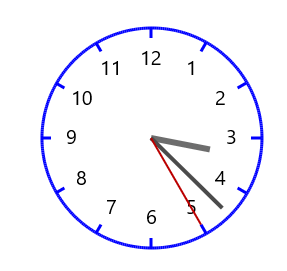1. It uses two layers, one for the clock layout and one for the hands. Only the second layer is redrawn every second.
2. It uses a timer that ticks every second.
3. Turtle is set to be in "rabbit mode" - there are no animations.
4. The turtle itself is hidden with SetTurtleVisible (False).

Code:

B4X:
``Private Timer1 As Timer``
2.:
B4X:
``````Sub Turtle_Start
If Timer1.IsInitialized = False Then
Timer1.Initialize("Timer1", 1000)
End If
Turtle.RabbitMode.SetTurtleVisible(False)
Turtle.SetPenColor(xui.Color_Blue).SetPenSize(3)
Turtle.SetNumberOfLayers(2)
Turtle.SetCurrentLayer(0).ClearScreen
Turtle.TurnRight(90).PenUp.MoveForward(110).PenDown.TurnLeft(90)
DrawCircle(110)
For i = 1 To 12
Turtle.Home
Turtle.SetAngle(HoursToAngle(i))
Turtle.SetPenColor(xui.Color_Blue)
Turtle.PenUp.MoveForward(100).PenDown.MoveForward(10).PenUp.MoveBackward(30)
Turtle.SetPenColor(xui.Color_Black).DrawText(i)
Next
Timer1.Enabled = True
End Sub

Sub Timer1_Tick
Turtle.SetCurrentLayer(1) 'second layer
Turtle.ClearScreen
Turtle.Home
Dim hours As Int = DateTime.GetHour(DateTime.Now)
Dim minutes As Int = DateTime.GetMinute(DateTime.Now)
Dim seconds As Int = DateTime.GetSecond(DateTime.Now)
Turtle.PenDown
Turtle.SetPenSize(6).SetPenColor(0xFF6D6D6D)
Turtle.SetAngle(HoursToAngle(hours + minutes / 60)).MoveForward(60)
Turtle.SetPenSize(4).SetPenColor(0xFF4B4B4B).Home
Turtle.SetAngle(MinutesOrSecondsToAngle(minutes + seconds / 60)).MoveForward(100)
Turtle.SetPenSize(2).SetPenColor(0xFFBB0000).Home
Turtle.SetAngle(MinutesOrSecondsToAngle(seconds)).MoveForward(100)
End Sub

Sub HoursToAngle (Hours As Float) As Float
Return Hours / 12 * 360 - 90
End Sub

Sub MinutesOrSecondsToAngle (N As Float) As Float
Return N / 60 * 360 - 90
End Sub

Dim DistancePerIteration As Float = 2 * cPI * Radius / 360
For i = 1 To 360
Turtle.MoveForward(DistancePerIteration).TurnLeft(1)
Next
End Sub

Sub MainForm_Resize (Width As Double, Height As Double)
If Timer1.IsInitialized = False Then Return 'Resize event can be raised before Turtle_Start. We want to ignore those events.
Turtle.Stop.ClearScreen.Home
Turtle_Start
End Sub``````
Depends on B4XTurtle 1.03+.

••HamedBagheri, soyVB6, maXim and 8 others

#### Erel

Staff member
Rabbit mode and the Get methods. This is an important example.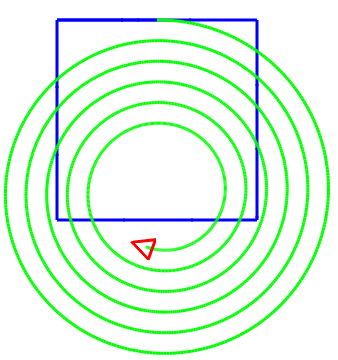The code:
B4X:
``````Turtle.SetPenColor(xui.Color_Blue).SetPenSize(3).SetSpeedFactor(20)
Turtle.SetX(100).SetY(100)
For i = 1 To 4
Turtle.MoveForward(200).TurnRight(90)
Next
Turtle.MoveForward(100).SetPenColor(xui.Color_Green)
For i = 1 To 2000
Turtle.MoveForward(3 - i / 1000)
Turtle.TurnRight(1)
Next``````
Now we want to limit the spiral drawing to the square interior.
So we add code that raises or puts down the pen based on the values returned from Turtle.GetX and Turtle.GetY:
B4X:
``````For i = 1 To 2000
Dim x As Float = Turtle.GetX
Dim y As Float = Turtle.GetY
If x >= 100 And x <= 300 And y >= 100 And y <= 300 Then
Turtle.PenDown
Else
Turtle.PenUp
End If
Turtle.MoveForward(3 - i / 1000)
Turtle.TurnRight(1)
Next``````
It doesn't work. The full spiral is drawn, exactly as above.

So we add a call to Log inside the loop that will print the coordinates in the logs tab:
B4X:
``Log("x = " & x & ", y = " & y)``
Surprisingly it prints: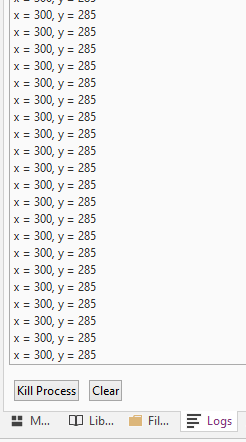These are the "home" coordinates.

Why GetX and GetY return the wrong values???

The answer is that the actions are not executed immediately, they are added to a queue and the queue is then processed. This allows the drawings to be animated.
So all of this code will be executed before the turtle moves a single pixel. This is why GetX and GetY return the home coordinates.

The solution is to switch to rabbit mode. In rabbit mode the actions are executed immediately.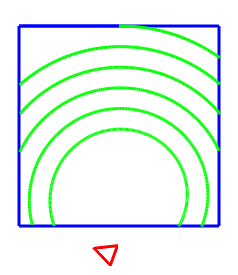The complete code:
B4X:
``````Sub Turtle_Start
Turtle.RabbitMode
Turtle.SetPenColor(xui.Color_Blue).SetPenSize(3).SetSpeedFactor(20)
Turtle.SetX(100).SetY(100)
For i = 1 To 4
Turtle.MoveForward(200).TurnRight(90)
Next
Turtle.MoveForward(100).SetPenColor(xui.Color_Green)
For i = 1 To 2000
Dim x As Float = Turtle.GetX
Dim y As Float = Turtle.GetY
Log("x = " & x & ", y = " & y)
If x >= 100 And x <= 300 And y >= 100 And y <= 300 Then
Turtle.PenDown
Else
Turtle.PenUp
End If
Turtle.MoveForward(3 - i / 1000)
Turtle.TurnRight(1)
Next
End Sub``````
Note that it is important that RabbitMode will be the very first call.

If the call to RabbitMode is not the first call, it will only switch to rabbit mode when the action is processed. This will happen after the code is executed and again the Get methods will not return the expected values.
The way to switch to rabbit mode and to be able to get the current values is by waiting for the Done event:
B4X:
``````Sub DrawSpiralInsideSquare
If Turtle.IsMoving Then 'important check. If IsMoving is False then the Done event will not be raised.
Wait For Turtle_Done
End If
Turtle.RabbitMode
Turtle.MoveForward(100).SetPenColor(xui.Color_Green)
For i = 1 To 2000
Dim x As Float = Turtle.GetX
Dim y As Float = Turtle.GetY
If x >= 100 And x <= 300 And y >= 100 And y <= 300 Then
Turtle.PenDown
Else
Turtle.PenUp
End If
Turtle.MoveForward(3 - i / 1000)
Turtle.TurnRight(1)
Next
End Sub``````

•maXim and Johan Hormaza

#### Erel

Staff member
Drawing bitmaps:B4X:
``````Sub Turtle_Start
Turtle.SetPenColor(xui.Color_Blue).SetPenSize(3).SetSpeedFactor(1)
Dim smiley As B4XBitmap = xui.LoadBitmapResize(File.DirAssets, "smiley.png", 100, 100, True)
Turtle.PenUp
For i = 0 To 360
Turtle.MoveForward(2).TurnLeft(1)
If i Mod 60 = 0 Then Turtle.DrawBitmap(smiley)
Next
End Sub``````
Add the attached file to the Files tab.

#### Attachments

• 3.8 KB Views: 16
•maXim and Johan Hormaza

#### Erel

Staff member
PushState / PopState

We want to draw this nice circle:B4X:
``````Sub Turtle_Start
Turtle.SetPenColor(xui.Color_Blue).SetPenSize(3).SetSpeedFactor(1)
For i = 1 To 360
Turtle.MoveForward(2).TurnLeft(1)
If i Mod 60 = 0 Then DrawGreenCircle
Next
End Sub

Sub DrawGreenCircle
Turtle.SetPenColor(xui.Color_Green)
For i = 1 To 360
Turtle.MoveForward(0.5).TurnLeft(1)
Next
End Sub``````
Worth explaining line #5, the Mod operator (modulo) is a very important operator. It returns the remainder of i divided by 60. It will be 0 when 'i' equals to: 0, 60, 120, 180 and so on.
The result is that we call DrawGreenCircle every 60 degrees. (it would have been a bit more elegant, at least for myself, if the for loop range was from 0 to 359 but it is not important).

We run the code and we get: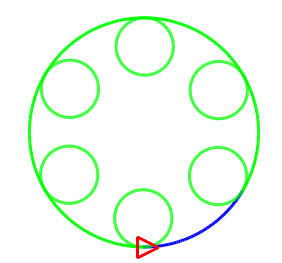There is a big problem. Ideally, a sub (method) shouldn't have side effects. We want to call it, let it do its job, and continue with our job. This is not the case here as it changed the pen color.
BTW, this is one of the reasons that you should use at least as possible global variables. A sub that modifies global variables is a sub that has side effects and is therefore a sub that adds complexity to your program.

The solution with B4XTurtle is simple.

We change the sub code to:
B4X:
``````Sub DrawGreenCircle
Turtle.PushState
Turtle.SetPenColor(xui.Color_Green)
For i = 1 To 360
Turtle.MoveForward(0.5).TurnLeft(1)
Next
Turtle.PopState
End Sub``````
When it starts it "pushes" the current turtle state to an internal stack. Right before it ends it pops the newly added state and returns the turtle state to the previous one.
A stack is a Last In First Out (LIFO) data collection. The last state that was added is the first one to be removed. This is different than a queue which is a First In First Out (FIFO) data collection.

Last edited:

#### Erel

Staff member
Multilayers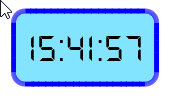By default there is a single drawing layer, however B4XTurtle supports multiple layers. In this example the background is drawn on layer #0 and the time string is drawn on the second layer which is layer #1. Indices in B4X always start from 0.
SetNumberOfLayers defines the number of layers.
SetCurrentLayer sets the current layer.

I've used a custom font here. Source: https://www.dafont.com/ds-digital.font
The code to load custom fonts is platform specific. This is the B4J code. It is quite similar in the other platforms.
B4X:
``````Sub Turtle_Start
Turtle.SetNumberOfLayers(2)
Turtle.SetCurrentLayer(0) 'first layer = 0
Turtle.SetPenColor(xui.Color_Blue).SetPenSize(5).SetSpeedFactor(2)
Turtle.SetX(40).SetY(125)
For i = 1 To 2
Turtle.MoveForward(120)
RoundCorner
Turtle.MoveForward(50)
RoundCorner
Next
Turtle.PenUp.TurnLeft(90).MoveForward(20).SetPenColor(0xFF82E4FF).Fill
Turtle.SetCurrentLayer(1) 'switch to second layer
If Timer1.IsInitialized = False Then
Timer1.Initialize("Timer1", 1000)
Timer1.Enabled = True
End If
Turtle.SetTurtleVisible(False)
End Sub

Sub RoundCorner
For i = 1 To 90
Turtle.MoveForward(0.2).TurnLeft(1)
Next
End Sub

Sub Timer1_Tick
Turtle.ClearScreen
Turtle.SetPenColor(xui.Color_Black)
Turtle.SetX(100).SetY(90).DrawText(DateTime.Time(DateTime.Now))
End Sub``````
B4X:
``Private Timer1 As Timer``

•Johan Hormaza and maXim

#### Erel

Staff member
Lists and For loops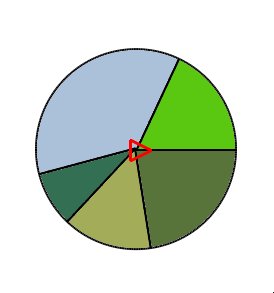List is a simple and important data structure. You can add and remove elements from a list. The elements can be of any type.
B4X:
``````Sub Turtle_Start
Turtle.SetSpeedFactor(3)
Dim values As List
values.Initialize
DrawPie(values)
End Sub``````
There are two common ways to iterate over the list items:

1. For Each:
B4X:
``````For Each value As Float In Values
'do something here
Next``````
2. For
B4X:
``````For i = 0 To Values.Size - 1 'first time index is always 0
Dim value As Float = Values.Get(i)
'do something here
Next``````
For Each is safer as you cannot get the index wrong. As a general rule you should use For Each unless you need to access the index.

If you are familiar with arrays, then a list is a dynamic array. It can grow and shrink as needed.
In B4X, arrays can be converted to lists automatically. This allows us to replace the above code with:
B4X:
``DrawPie (Array(100, 200, 50, 80, 120))``
Complete code:
B4X:
``````Sub Turtle_Start
Turtle.SetSpeedFactor(3)
Dim values As List
values.Initialize
DrawPie(values)
End Sub

Sub DrawPie (Values As List)
Dim radius As Float = 100
Dim TotalValues As Float
'For Each - best option when we don't need the index
For Each value As Float In Values
TotalValues = TotalValues + value
Next
Dim TotalAngles As Float
'regular For Next
For i = 0 To Values.Size - 1 'first time index is always 0
Dim value As Float = Values.Get(i)
Dim angle As Float = Floor(value / TotalValues * 360)
If i = Values.Size - 1 Then
'to avoid rounding errors the last element completes the circle.
angle = Ceil(360 - TotalAngles)
End If
TotalAngles = TotalAngles + angle
Next
End Sub

Sub DrawSlice (angle As Float, radius As Float)